# How to calculate weighted average in an Excel Pivot Table?

Using a combination of Excel's SUMPRODUCT and SUM functions, the weighted average may be quickly and easily calculated. However, it appears that the functions in a pivot table are not supported by the calculated fields. As a result, how exactly would one go about calculating the weighted average in a pivot table? This article will present a solution to the problem.

## Calculating Weighted Average in an Excel Pivot Table

Let’s understand step by step with an example.

### Step 1

In the first step, we must have a sample data for creating pivot table as shown in the below screenshot for the same.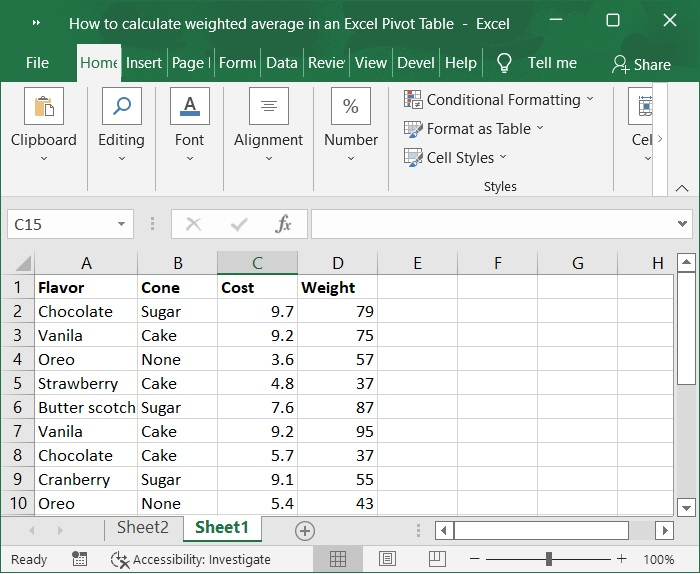### Step 2

Now, select the data range A1:D10. Click on the Insert tab on the toolbar ribbon and then select Pivot table option to insert pivot table for the selected data range. Refer to the below screenshot for the same.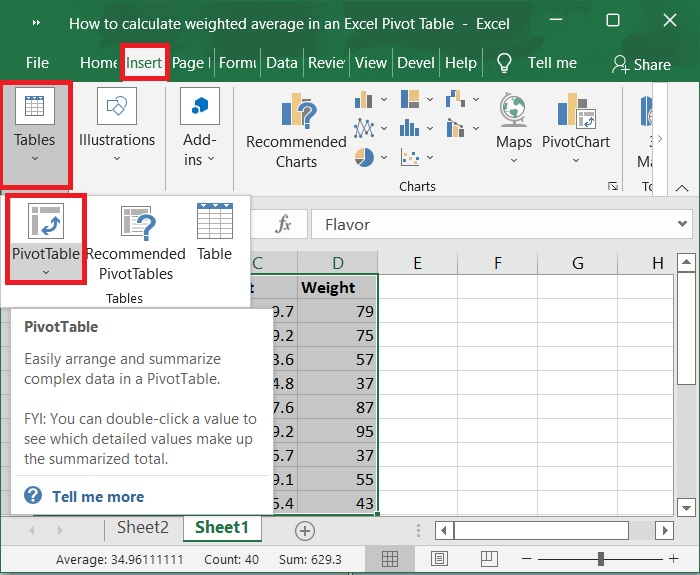### Step 3

In the next step, create pivot table window appears, make sure the data range is selected as A1:D10 under select table/range option. Now, choose new worksheet to create the pivot table in separate sheet then click on OK button. Below screenshot for the same.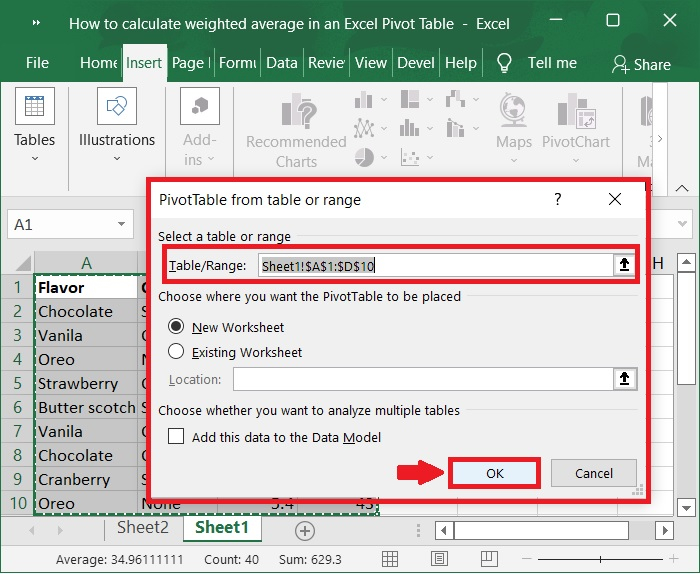### Step 4

The pivot table is now created in separate worksheet as shown in the below screenshot for same.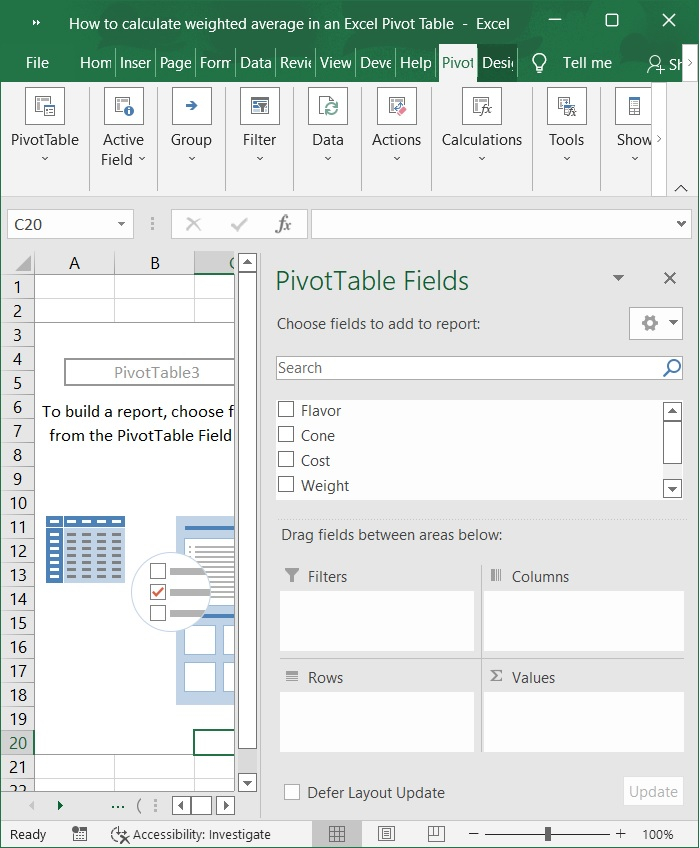### Step 5

In the Pivot table fields check flavor, cost and weight. After those values appear. Please refer to the below screenshot for the same.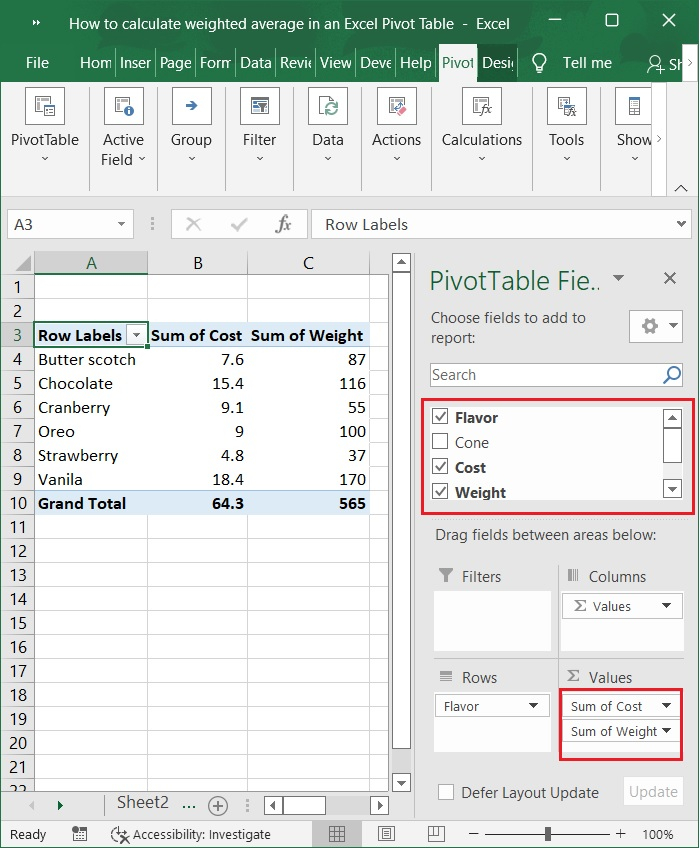In order to demonstrate how to calculate the weighted average price of each flavor in the pivot table, I will use the pivot table as an example.

### Step 6

Next, add an Average helper column to the source data.

Create a new, empty column in the source data, name it Average, enter the below formula in the first cell of this helper column, and then drag the AutoFill handle to fill the entire column. Please check out the below screenshot for the same.

=D2*E2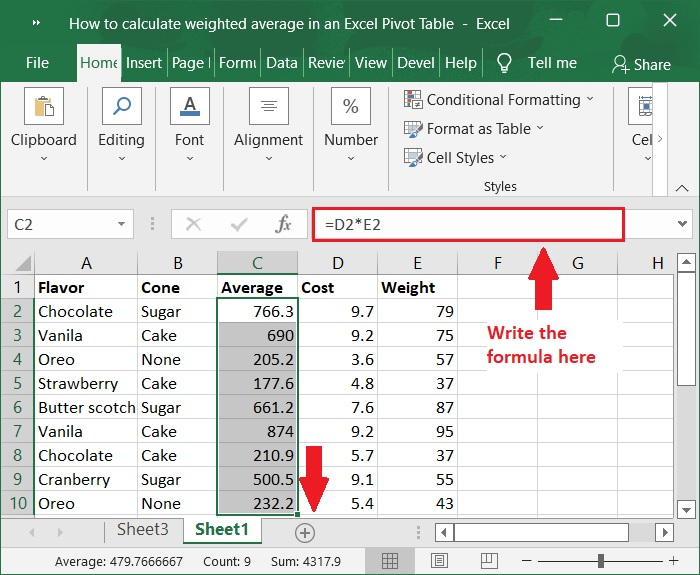### Step 7

To access the PivotTable Tools, choose any cell in the pivot table, and then click Pivot Analyze (or Options) then select Refresh option. Please refer to the below screenshot for the same.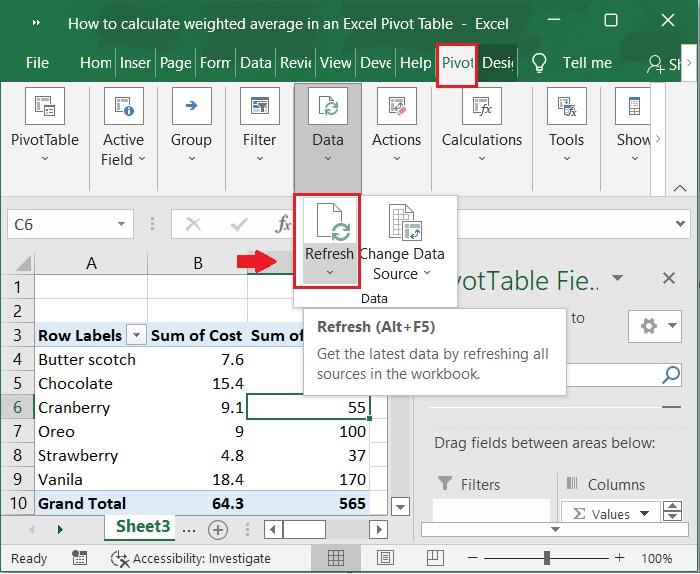### Step 8

After that, in pivot table tools select Analyze option and click Fields, items, & sets then choose calculated Field. Please check out the below screenshot.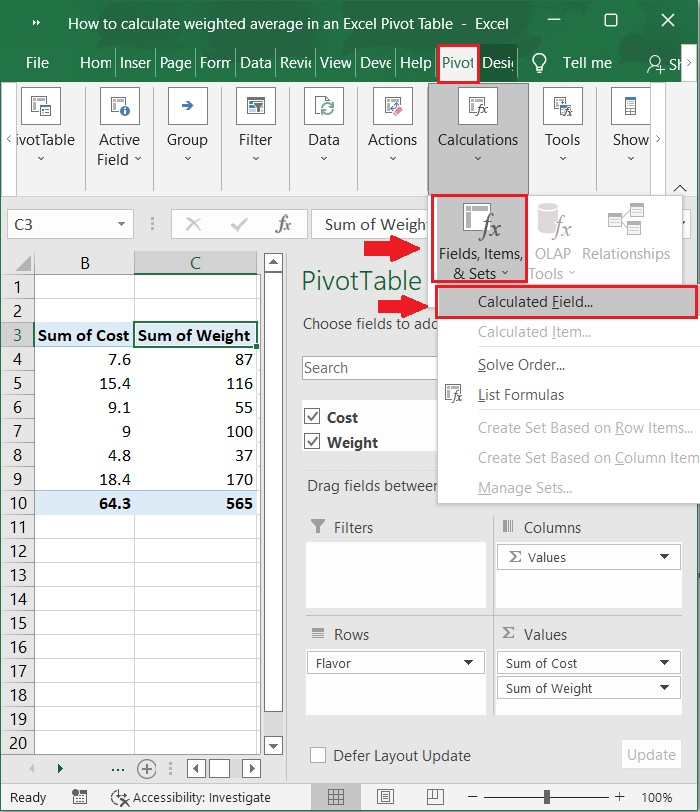### Step 9

Then, the Insert Calculated Field dialog box appeared, type Weighted Average in the Name box, and in the Formula box type =Average/Weight (please change the formula based on the names of your fields), and then click the OK button to close the dialog box. Please check out the screenshot below for the same.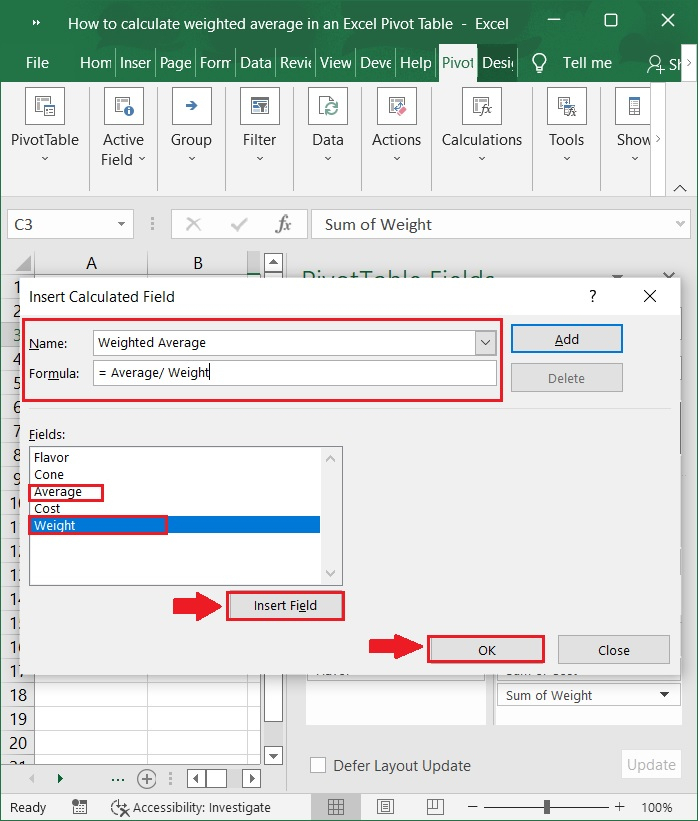### Step 10

Once you return to the pivot table at this point, you will see that the subtotal rows now contain the weighted average price of each flavor. Below screenshots for the same.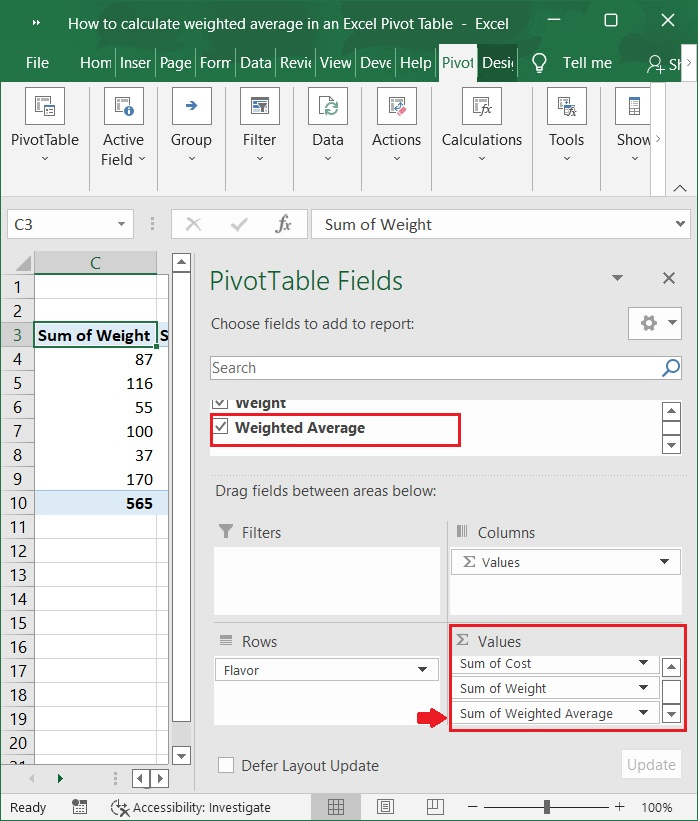The final screen will appear as the one shown below −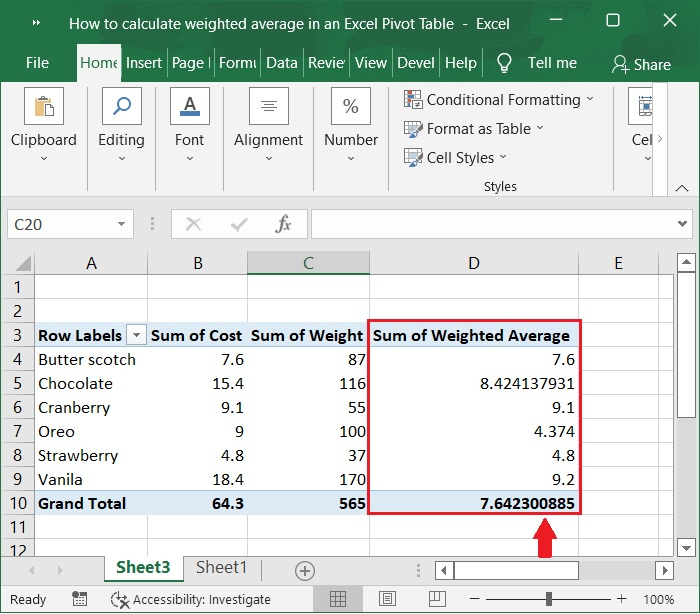## Conclusion

In this tutorial, we used a simple example to demonstrate how you can calculate weighted average in an Excel pivot table.